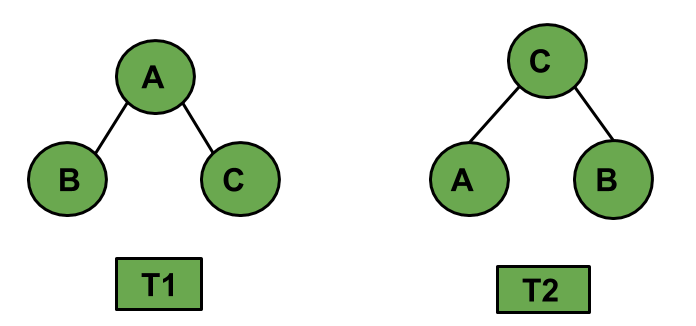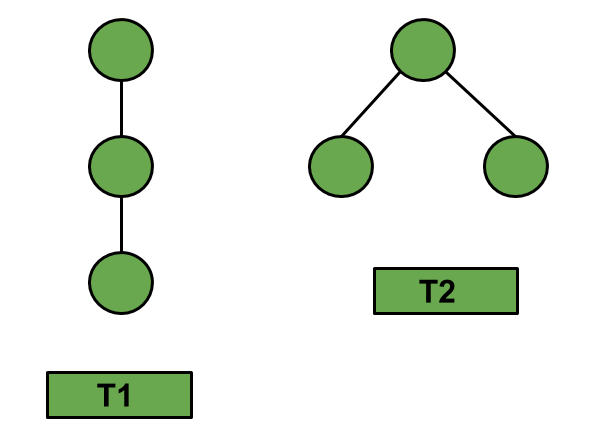# Combinatorics on ordered trees

An ordered tree is an oriented tree in which the children of a node are somehow ordered. It is a rooted tree in which an ordering is specified for the children of each vertex. This is called a “plane tree” because an ordering of the children is equivalent to an embedding of the tree in the plane, with the root at the top and the children of each vertex lower than that vertex.
Ordered tree can be further specified as labelled ordered tree and unlabelled ordered tree.

Prerequisite : Catalan Numbers | Binomial Coefficient.

Labelled ordered trees : A labeled tree is a tree where each vertex is assigned a unique number from 1 to n.If T1 and T2 are ordered trees. Then, T1 != T2 else T1 = T2.

Unlabelled ordered trees : An unlabelled tree is a tree where every vertex is unlabelled. Given below are the possible unlabelled ordered tree having 3 vertices.The total number of unlabelled ordered trees having n nodes is equal to the (n – 1)-th Catalan Number.

Given below are the possible unlabelled ordered trees having 4 nodes. This diagram will work as a reference example for the next few results.1. Number of trees with exactly k leaves.

Let us consider, we have a ‘n’ edges . Then, the solution for the total possible ordered trees having ‘k’ leaves is given by :2. Total number of nodes of degree d in these trees.

Let us consider, we have a ‘n’ edges . Then, the solution for the total number of nodes having degree ‘d’ is given by :3. Number of trees in which the root has degree r.

Let us consider, we have a ‘n’ edges . Then, the solution for the total possible ordered trees whose root has degree ‘r’ is given by :Below is the implementation of above combinatorics functions using Binomial Coefficient :

## C++

 `// CPP code to find the number of ordered trees ` `// with given number of edges and leaves ` `#include ` `using` `namespace` `std; ` ` `  `// Function returns value of ` `// Binomial Coefficient C(n, k) ` `int` `binomialCoeff(``int` `n, ``int` `k) ` `{ ` `    ``int` `C[n + 1][k + 1] = { 0 }; ` `    ``int` `i, j; ` ` `  `    ``// Calculate value of Binomial ` `    ``// Coefficient in bottom up manner ` `    ``for` `(i = 0; i <= n; i++) { ` `        ``for` `(j = 0; j <= min(i, k); j++) { ` ` `  `            ``// Base Cases ` `            ``if` `(j == 0 || j == i) ` `                ``C[i][j] = 1; ` ` `  `            ``// Calculate value using ` `            ``// previously stored values ` `            ``else` `                ``C[i][j] = C[i - 1][j - 1] + C[i - 1][j]; ` `        ``} ` `    ``} ` ` `  `    ``return` `C[n][k]; ` `} ` ` `  `// Function to calculate the number ` `// of trees with exactly k leaves. ` `int` `k_Leaves(``int` `n, ``int` `k) ` `{ ` `    ``int` `ans = (binomialCoeff(n, k) * binomialCoeff(n, k - 1)) / n; ` `    ``cout << ``"Number of trees having 4 edges"` `         ``<< ``" and exactly 2 leaves : "` `<< ans << endl; ` `    ``return` `0; ` `} ` ` `  `// Function to calculate total number of ` `// nodes of degree d in these trees. ` `int` `numberOfNodes(``int` `n, ``int` `d) ` `{ ` `    ``int` `ans = binomialCoeff(2 * n - 1 - d, n - 1); ` `    ``cout << ``"Number of nodes of degree 1 in"` `         ``<< ``" a tree having 4 edges : "` `<< ans << endl; ` `    ``return` `0; ` `} ` ` `  `// Function to calculate the number of ` `// trees in which the root has degree r. ` `int` `rootDegreeR(``int` `n, ``int` `r) ` `{ ` `    ``int` `ans = r * binomialCoeff(2 * n - 1 - r, n - 1); ` `    ``ans = ans / n; ` `    ``cout << ``"Number of trees having 4 edges"` `         ``<< ``" where root has degree 2 : "` `<< ans << endl; ` `    ``return` `0; ` `} ` ` `  `// Driver program to test above functions ` `int` `main() ` `{ ` `    ``// Number of trees having 3 ` `    ``// edges and exactly 2 leaves ` `    ``k_Leaves(3, 2); ` ` `  `    ``// Number of nodes of degree ` `    ``// 3 in a tree having 4 edges ` `    ``numberOfNodes(3, 1); ` ` `  `    ``// Number of trees having 3 ` `    ``// edges where root has degree 2 ` `    ``rootDegreeR(3, 2); ` ` `  `    ``return` `0; ` `} `

## Java

 `// java code to find the number of ordered  ` `// trees with given number of edges and ` `// leaves ` `import` `java.io.*; ` ` `  `class` `GFG { ` `         `  `    ``// Function returns value of ` `    ``// Binomial Coefficient C(n, k) ` `    ``static` `int` `binomialCoeff(``int` `n, ``int` `k) ` `    ``{ ` `        ``int` `[][]C = ``new` `int``[n+``1``][k+``1``]; ` `        ``int` `i, j; ` `     `  `        ``// Calculate value of Binomial ` `        ``// Coefficient in bottom up manner ` `        ``for` `(i = ``0``; i <= n; i++) { ` `            ``for` `(j = ``0``; j <= Math.min(i, k); j++) ` `            ``{ ` `     `  `                ``// Base Cases ` `                ``if` `(j == ``0` `|| j == i) ` `                    ``C[i][j] = ``1``; ` `     `  `                ``// Calculate value using ` `                ``// previously stored values ` `                ``else` `                    ``C[i][j] = C[i - ``1``][j - ``1``]  ` `                               ``+ C[i - ``1``][j]; ` `            ``} ` `        ``} ` `     `  `        ``return` `C[n][k]; ` `    ``} ` `     `  `    ``// Function to calculate the number ` `    ``// of trees with exactly k leaves. ` `    ``static` `int` `k_Leaves(``int` `n, ``int` `k) ` `    ``{ ` `        ``int` `ans = (binomialCoeff(n, k) * ` `                ``binomialCoeff(n, k - ``1``)) / n; ` `        ``System.out.println( ``"Number of trees "` `             ``+ ``"having 4 edges and exactly 2 "` `                        ``+ ``"leaves : "` `+ ans) ; ` `        ``return` `0``; ` `    ``} ` `     `  `    ``// Function to calculate total number of ` `    ``// nodes of degree d in these trees. ` `    ``static` `int` `numberOfNodes(``int` `n, ``int` `d) ` `    ``{ ` `        ``int` `ans = binomialCoeff(``2` `* n - ``1` `- d, ` `                                       ``n - ``1``); ` `        ``System.out.println(``"Number of nodes "` `           ``+``"of degree 1 in a tree having 4 "` `                          ``+ ``"edges : "` `+ ans); ` `        ``return` `0``; ` `    ``} ` `     `  `    ``// Function to calculate the number of ` `    ``// trees in which the root has degree r. ` `    ``static` `int` `rootDegreeR(``int` `n, ``int` `r) ` `    ``{ ` `        ``int` `ans = r * binomialCoeff(``2` `* n  ` `                              ``- ``1` `- r, n - ``1``); ` `        ``ans = ans / n; ` `        ``System.out.println(``"Number of trees "` `            ``+ ``"having 4 edges where root has"` `                      ``+ ``" degree 2 : "` `+ ans); ` `        ``return` `0``; ` `    ``} ` `     `  `    ``// Driver program to test above functions ` ` `  `    ``public` `static` `void` `main (String[] args) ` `    ``{ ` `             `  `        ``// Number of trees having 3 ` `        ``// edges and exactly 2 leaves ` `        ``k_Leaves(``3``, ``2``); ` `     `  `        ``// Number of nodes of degree ` `        ``// 3 in a tree having 4 edges ` `        ``numberOfNodes(``3``, ``1``); ` `     `  `        ``// Number of trees having 3 ` `        ``// edges where root has degree 2 ` `        ``rootDegreeR(``3``, ``2``); ` `    ``} ` `} ` ` `  `// This code is contributed by anuj_67. `

## C#

 `// C# code to find the number of ordered  ` `// trees with given number of edges and ` `// leaves ` `using` `System; ` ` `  `class` `GFG { ` `         `  `    ``// Function returns value of ` `    ``// Binomial Coefficient C(n, k) ` `    ``static` `int` `binomialCoeff(``int` `n, ``int` `k) ` `    ``{ ` `        ``int` `[,]C = ``new` `int``[n+1,k+1]; ` `        ``int` `i, j; ` `     `  `        ``// Calculate value of Binomial ` `        ``// Coefficient in bottom up manner ` `        ``for` `(i = 0; i <= n; i++) { ` `            ``for` `(j = 0; j <= Math.Min(i, k); j++) ` `            ``{ ` `     `  `                ``// Base Cases ` `                ``if` `(j == 0 || j == i) ` `                    ``C[i,j] = 1; ` `     `  `                ``// Calculate value using ` `                ``// previously stored values ` `                ``else` `                    ``C[i,j] = C[i - 1,j - 1]  ` `                            ``+ C[i - 1,j]; ` `            ``} ` `        ``} ` `     `  `        ``return` `C[n,k]; ` `    ``} ` `     `  `    ``// Function to calculate the number ` `    ``// of trees with exactly k leaves. ` `    ``static` `int` `k_Leaves(``int` `n, ``int` `k) ` `    ``{ ` `        ``int` `ans = (binomialCoeff(n, k) * ` `                ``binomialCoeff(n, k - 1)) / n; ` `        ``Console.WriteLine( ``"Number of trees "` `            ``+ ``"having 4 edges and exactly 2 "` `                        ``+ ``"leaves : "` `+ ans) ; ` `        ``return` `0; ` `    ``} ` `     `  `    ``// Function to calculate total number of ` `    ``// nodes of degree d in these trees. ` `    ``static` `int` `numberOfNodes(``int` `n, ``int` `d) ` `    ``{ ` `        ``int` `ans = binomialCoeff(2 * n - 1 - d, ` `                                    ``n - 1); ` `        ``Console.WriteLine(``"Number of nodes "` `        ``+``"of degree 1 in a tree having 4 "` `                        ``+ ``"edges : "` `+ ans); ` `        ``return` `0; ` `    ``} ` `     `  `    ``// Function to calculate the number of ` `    ``// trees in which the root has degree r. ` `    ``static` `int` `rootDegreeR(``int` `n, ``int` `r) ` `    ``{ ` `        ``int` `ans = r * binomialCoeff(2 * n  ` `                            ``- 1 - r, n - 1); ` `        ``ans = ans / n; ` `        ``Console.WriteLine(``"Number of trees "` `            ``+ ``"having 4 edges where root has"` `                    ``+ ``" degree 2 : "` `+ ans); ` `        ``return` `0; ` `    ``} ` `     `  `    ``// Driver program to test above functions ` ` `  `    ``public` `static` `void` `Main () ` `    ``{ ` `             `  `        ``// Number of trees having 3 ` `        ``// edges and exactly 2 leaves ` `        ``k_Leaves(3, 2); ` `     `  `        ``// Number of nodes of degree ` `        ``// 3 in a tree having 4 edges ` `        ``numberOfNodes(3, 1); ` `     `  `        ``// Number of trees having 3 ` `        ``// edges where root has degree 2 ` `        ``rootDegreeR(3, 2); ` `    ``} ` `} ` ` `  `// This code is contributed by anuj_67. `

## PHP

 ` `

Output:

```Number of trees having 4 edges and exactly 2 leaves : 3
Number of nodes of degree 1 in a tree having 4 edges : 6
Number of trees having 4 edges where root has degree 2 : 2
```

Time Complexity : O(n*k).
Auxiliary Space : O(n*k).My Personal Notes arrow_drop_upCheck out this Author's contributed articles.

If you like GeeksforGeeks and would like to contribute, you can also write an article using contribute.geeksforgeeks.org or mail your article to contribute@geeksforgeeks.org. See your article appearing on the GeeksforGeeks main page and help other Geeks.

Please Improve this article if you find anything incorrect by clicking on the "Improve Article" button below.

Improved By : vt_m, nidhi_biet

1

Please write to us at contribute@geeksforgeeks.org to report any issue with the above content.